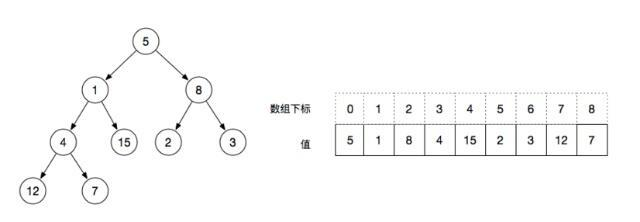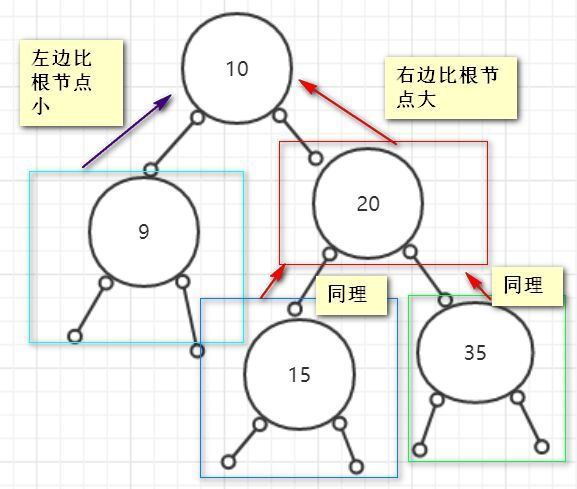讲透学烂二叉树 (四)：二叉树的存储结构—建堆 - 搜索 - 排序zhoulujun

二叉树的存储结构

二叉树存储方式

顺序存储结构1. Left = index * 2 + 1，例如：根节点的下标为 0，则左节点的值为下标 array[0＊2+1]=1

2. Right = index * 2 + 2，例如：根节点的下标为 0，则右节点的值为下标 array[0＊2+2]=2

3. Parent(i) = floor((i-1)/2)，i 的父节点下标，Math.ceil((index/2)-1（向上取整），其父节点下标为 floor((i-1)/2)（向下取整）

4. 序数 >= floor(N/2)都是叶子节点，例如：floor(9/2) = 4，则从下标 4 开始的值都为叶子节点

顺序存储结构 JavaScript 代码演示

数组转化二叉树
/** * @name 层序遍历 * @description * left = index * 2 + 1，例如：根节点的下标为0，则左节点的值为下标array[0＊2+1]=1 * right = index * 2 + 2，例如：根节点的下标为0，则右节点的值为下标array[0＊2+2]=2 * 序数 >= floor(N/2)都是叶子节点，例如：floor(9/2) = 4，则从下标4开始的值都为叶子节点 * index>0，index的结点的父节点为Math.ceil((index/2)-1， * @param arr {number} * @return {null|[]} */buildTreeByLevelOrder (arr) {    let nodesList = this.createNodeList(arr)    let length = nodesList.length    if (length === 0) {        return null    }    // 确定每个结点的左右结点    for (let i = 0; i < length / 2 - 1; i++) {        if (2 * i + 1 < length) {            nodesList[i].leftChild = nodesList[2 * i + 1]            nodesList[i].parentNode = nodesList[Math.floor((i - 1) / 2)]        }        if (2 * i + 2 < length) {            nodesList[i].rightChild = nodesList[2 * i + 2]            nodesList[i].parentNode = nodesList[Math.floor((i - 1) / 2)]        }    }    // 判断最后一个根结点：因为最后一个根结点可能没有右结点，所以单独拿出来处理    let lastIndex = Math.round(length / 2) - 1    // 左结点    nodesList[lastIndex].leftChild = nodesList[lastIndex * 2 + 1]    // 右结点，如果数组的长度为奇数才有右结点    if (length % 2 === 1) {        nodesList[lastIndex].rightChild = nodesList[lastIndex * 2 + 2]    }    this.tree = nodesList    return nodesList}

二叉树层转化为数组(层序遍历）
/** * @description 深度优先递归 * @param tree {Node} * @return {Array} */levelTraverse (tree) {    // 分层，每层放一个数组    let buckets = [[tree]]    let i = 0    // 递归，    function tempFunction (node, i) {        i++        let nodes = []        if (node.leftChild) {            nodes.push(node.leftChild)        }        if (node.rightChild) {            nodes.push(node.rightChild)        }        if (nodes.length) {            if (buckets[i] === undefined) {                buckets[i] = nodes            } else {                buckets[i].push(...nodes)            }            nodes.forEach(item => {                tempFunction(item, i)            })        }    }    tempFunction(tree, 0)    let backs = []    buckets.forEach(bucket=>{        backs.push(...bucket.map((node)=>node.data))    })    return  backs}

搜索树的链式存储结构

• 一个指向父亲节点的指针 parent

• 一个指向左节点的指针 left

• 一个指向右节点的指针 right

• 一个数据元素，里面可以是一个 key 和 value

class BinaryTreeNode {    constructor (key, value) {        this.parent = null;        this.left = null;        this.right = null;        this.key = key;        this.value = value;    }}

链式存储结构 JavaScript 代码演示

数组转换为二叉-先序遍历构建二叉树/** * @description 先序遍历构建二叉树 * @param arr */buildBinaryTreeByPreOrder (arr) {    let root = new Node(arr.shift())    arr.forEach(data => {        this.insertNumber(data, root)    })    this.tree = root    return root}
/** * @description 插入节点 * @param data {Number} 节点值，暂时为数字 * @param tree {Node} 插入的树 */insertNumber (data, tree = this.tree) {    let newNode = new Node(data)    debugger    if (!tree.data) {        tree.data = data    } else {        this.insertNode(tree, newNode)    }}
/** * @description 插入节点 * @param node {Node} 节点值，暂时为数字 * @param newNode {Node} 插入的树 */insertNode (node, newNode) {    newNode.parentNode = node    if (newNode.data < node.data) {        if (node.leftChild === null) {            node.leftChild = newNode        } else {            this.insertNode(node.leftChild, newNode)        }    } else {        if (node.rightChild === null) {            node.rightChild = newNode        } else {            this.insertNode(node.rightChild, newNode)        }    }}

二叉树转换为数组
/** * @description 前序遍历 =>1.访问根节点； 2.访问左子树； 3.访问右子树 * @param node {Node} 遍历的树 */preOrderTraverse (node = this.tree) {    // 数组存储数遍历值    let backs = []
// 递归，    function tempFunction (node) {        if (node.data !== null) {            // 先取根结点            backs.push(node.data)            // 如果存在左结点，取左节点的值            node.leftChild && tempFunction(node.leftChild)            // 如果存在右结点，取右结点值            node.rightChild && tempFunction(node.rightChild)        }    }
tempFunction(node)    return backs}

javascript/js 实现 排序二叉树数据结构 学习随笔 https://www.cnblogs.com/caimuguodexiaohongmao/p/11123933.html

js 中二叉树的深度遍历与广度遍历(递归实现与非递归实现) https://www.jianshu.com/p/5e9ea25a1aae评论